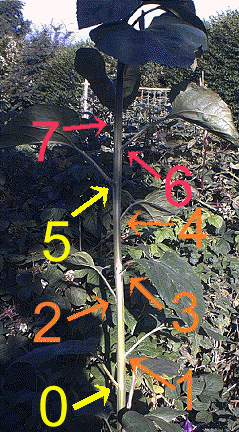See more about Fibonacci at our Fibonacci and Nature page. The Fibonacci Trick

 1 2 3 4 5 6 Total

• Ask a guest to choose any two numbers and write them in boxes 1 and 2. Hint: it makes life easier if the numbers are below 10!
• The guest then adds boxes 1 and 2, put the answer in box 3.
• Next they add boxes 2 and 3 and put the answer in box 4.
• Then they add boxes 3 and 4 and put the answer in box 5.
• Now YOU write a prediction on a separate piece of paper!
• The guest adds boxes 4 and 5 and puts the answer in box 6.
• The guest now adds up ALL the numbers in the six boxes and writes the answer in the "total" box.

Here's how it might look if the chosen numbers are 7 and 4:

 1 7 2 4 3 11 4 15 5 26 6 41 Total104

So how did you know the answer was going to be 104 even before box 6 was filled in? Answer - the prediction is 4 x the number in box 5! Here 4 x 26 =104.

The BIG Version!This trick and many others are explained in the book The PHANTOM X (algebra) There's more about Fibonacci in NUMBERS the Key to the Universe